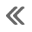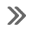#Honda Accord: Front Impact Sensor Replacement

Removal

1. Do t h e battery t e r m i n a l d i s c o n n e c t i o n procedure (see page 22-91), t h e n w a i t at least 3 m i n u t e s before s t a r t i ng work.

2. Remove t h e f r o n t b u m p e r (see page 20-255).

3. Left side: remove t h e intake air resonator (see page 11-333).

4. Disconnect t h e left or r i g h t engine c o m p a r t m e n t w i re harness 2P connector (A) f r o m t h e f r o n t impact sensor (B).5. Remove t h e TORX bolt (C) u s i n g a TORX T30 bit, t h en remove t h e f r o n t impact sensor.

Installation

1. Install t h e f r o n t impact sensor (A) w i t h n e w TORX bolts (B), u s i n g a TORX T30 bit. Connect t h e left or right engine c o m p a r t m e n t w i r e harness 2P connector (C) t o t h e f r o n t impact sensor.2. Do t h e battery t e r m i n a l reconnection procedure (see page 22-91).

3. Clear any DTCs w i t h t h e HDS (see page 24-38).

4. C o n f i rm proper SRS o p e r a t i o n : T u r n t h e i g n i t i on switch t o ON (II); t h e SRS indicator s h o u l d c o m e on f o r about 6 seconds and t h e n g o off.

5. Reinstall all r e m o v e d parts.

#####ODS Unit Replacement
Removal 1. Do t h e battery t e r m i n a l disconnection procedure (see page 22-91), t h e n w a i t at least 3 minutes before s t a r t i ng work. 2. Remove t h e f r o n t passenger's seat (s ...

#####Driver's Seat Position Sensor Replacement
Removal (Power seat) NOTE: Do not t u r n t h e i g n i t i o n s w i t c h t o ON (II), a n d do not connect t h e battery cable w h i l e r e m o v i n g t he d r i v e r ' s seat p o s i t i o n ...